# Precalculus : Find the Area of a Sector Using Radians

## Example Questions

### Example Question #381 : Pre Calculus

Find the area of a sector if the radius of the circle is 4, and the angle of the sector isradians.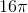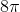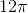Explanation:

Write the formula for the area of the sector in radians.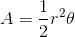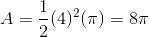### Example Question #382 : Pre Calculus

Find the area of a sector if the radius is 1 and the angle of sector isradians.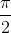Explanation:

Write the formula for the area of the sector in radians and substitute the given values.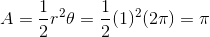### Example Question #1 : Find The Area Of A Sector Using Radians

If the diameter of a circle is 6, find the area of a sector with a sector angle of.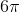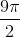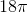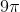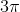Explanation:

Write the formula for the area of the sector in radians.The given diameter is 6, which means the radius is 3.

Substitute both the radius and theta to solve for the area.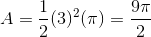### Example Question #384 : Pre Calculus

Find the area of a sector with the radius of 1 and angle of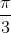.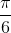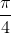Explanation:

Write the formula for the area of a sector in radians.Substitute the radius and the angle.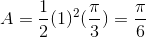### Example Question #385 : Pre Calculus

Find the area of a sector with a radius and angle of.  Express the answer in terms of.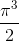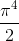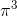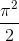Explanation:

Write the formula for the area of a sector.  Substitute the radius and angle to solve for the area.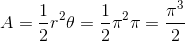### Example Question #386 : Pre Calculus

Find the area of a sector with a radius of 10 and an angle of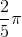radians.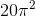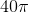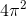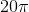Explanation:

Write the area of the sector in radians.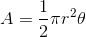Substitute the givens and solve.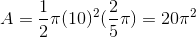### Example Question #31 : Angle Measures In Degrees And Radians

Katelyn is making a semi-circular design to put on one of her quilts. The design is not a perfect half-circle however, she needs to make the central angle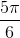radians. If the radius of the circle is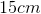, what is the area of the semi-circular design?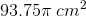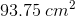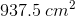Explanation:

Katelyn is making a semi-circular design to put on one of her quilts. The design is not a perfect half-circle however, she needs to make the central angleradians. If the radius of the circle is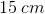, what is the area of the semi-circular design?

Recall the following formual for area of a sector: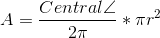So, we plug in our knowns and solve for area!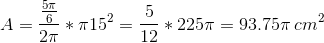### Example Question #388 : Pre Calculus

Lucy is making a solar panel to cover a portion of a satellite dish. If the central angle of the sector the solar panel will cover is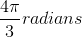, and the satellite dish has a radius of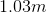, what area will the solar panel cover?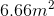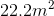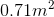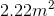Explanation:

Lucy is making a solar panel to cover a portion of a satellite dish. If the central angle of the sector the solar panel will cover is, and the satellite dish has a radius of, what area will the solar panel cover?

To calculate area of a sector, use the following formula: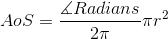Where the numerator of the fraction is the measure of the desired angle in radians, and r is the radius of the circle.

Now, we know both our variables, so we simply need to plug them in and simplify.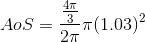Now, this looks messy, but we can simplify it to get: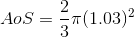Next, use your calculator to find a decimal answer, and then round to get our final answer.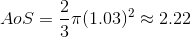Making the area of the sector### Example Question #389 : Pre Calculus

Find the area of a sector in a circle, given that it encompasses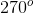of the actual circle, with a circle diameter of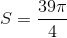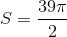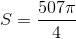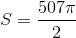Explanation:

Equation for sector area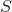is given by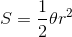, where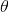is the angle measure of the sector in radians, andis the radius of the circle.

In our case, the sector encompasses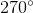of the circle or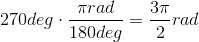To determine the radius, given diameter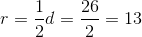The sector area therefore is: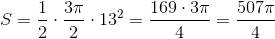### All Precalculus Resources